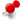# LibreCAD3 maths classesClassicListThreaded1 messageReply | Threaded
Open this post in threaded view
|

## LibreCAD3 maths classes

 I and Dli were discussing about the foundation of the mathematics portion of LibreCAD3, Regarding the linear and quadratic portions of the Solver. What I did was separated the Linear and the Quadratic portions, this way we were able to avoid most of the if else code that we do and implementations were separate for linear, quadratic and also linear/linear, linear/quadratic, quadratic/quadratic intersections finding. Dli has reviewed this, and has suggested that instead of separating the implementations, we should keep a single implementation ( quadratic one ) and when we are to calculate linear equations, we replace other values with 0. This way the if elses will be avoided but there might be some overkill when we will be calculating linear equations. I personally think separating implementations is better but that is some extra code to be maintained which in dli's suggestion is removed to some cost of useless calculations. So I'd like suggestions from others on this.